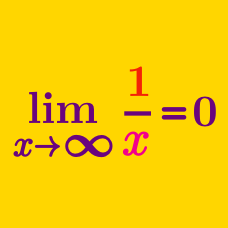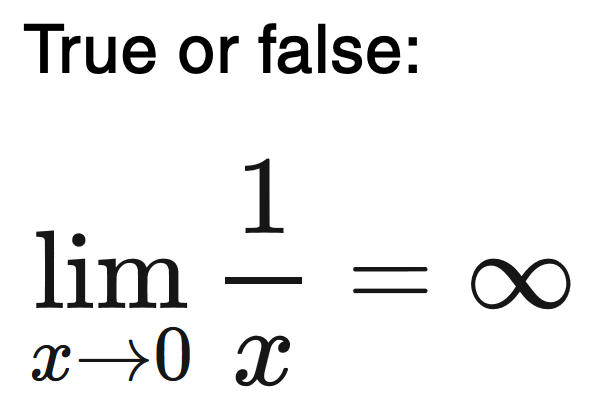Calculus

# Limits of Functions

## Intro

As $x$ gets closer and closer and closer, but never quite reaches its goal, how does your function behave?

Expect to see and learn how to solve questions like this one:(Be careful, most people who try this problem don’t get it right and/or get it right, but without understanding the full picture.)

Limits are used to describe the most extreme cases of a function’s behavior. They also allow you to use the “smoothness” of a function as a tool for understanding how a function behaves around strange singularities.

Being able to solve limit problems requires a combination of both knowing the essential strategies for simplifying functions and also understanding the rules for when limits do and don’t exist. For example, in the problem above, the limit does not exist because as x approaches 0 from the left, the values of $\frac{1}{x}$ approach $-\infty$ vs. as x approaches 0 from the right, the values of $\frac{1}{x}$ approach $\infty.$

In other cases, such as the $\lim_{x\to 0} \left|\frac{1}{x}\right|,$ there is no real, numerical limit as $\infty$ is not a real number, however, conceptually, we say that $\lim_{x\to 0} \left|\frac{1}{x}\right| = \infty,$ since, in this case, the left-hand and right-hand limits both equal $+\infty.$

Even the simplest-seeming limits questions trick many people because of how nuanced these tools and techniques are!

×